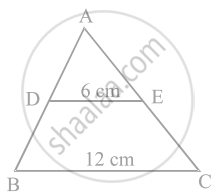# In figure, if DE || BC, find the ratio of ar(ΔADE) and ar (DECB). - Mathematics

Sum

In figure, if DE || BC, find the ratio of ar(ΔADE) and ar (DECB).#### Solution

Given, DE || BC, DE = 6 cm and BC = 12 cm

∠ACB = ∠AED   .....[Corresponding angle]

And ∠A = ∠A   .....[Common side]

∴ ΔABC ∼ ΔAED   .....[By AAA similarity criterion]

Then, (ar(ΔADE))/(ar(ΔABC)) = (DE)^2/(BC)^2

= (6)^2/(12)^2 = (1/2)^2

⇒ (ar(ΔADE))/(ar(ΔABC)) = (1/2)^2 = 1/4

Let ar(ΔADE) = k, then ar(ΔABC) = 4k

Now, ar(DECB) = ar(ABC) – ar(ΔADE) = 4k – k = 3k

∴ Required ratio = ar(ADE) : ar(DECB) = k : 3k = 1 : 3

Concept: Similarity of Triangles
Is there an error in this question or solution?

#### APPEARS IN

NCERT Mathematics Exemplar Class 10
Chapter 6 Triangles
Exercise 6.3 | Q 8 | Page 68

Share